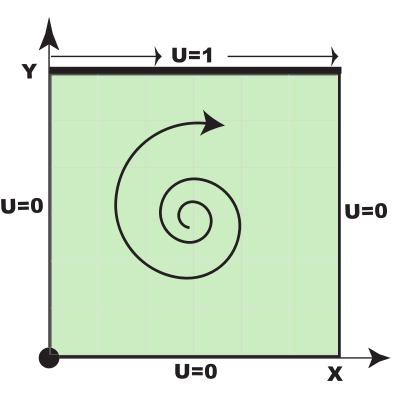Previous: Test case: Cavity Up: Test case: Cavity Next: Copying Tutorial

This is an automatically generated documentation by LaTeX2HTML utility. In case of any issue, please, contact us at info@cfdsupport.com.

## Physics

• Let us assume a two-dimensional flow in a cavity. Test case geometry is following:Figure: Test case geometry

• The fluid flow has following parameters:
• Top wall velocity: U = 1 m/s
• Cavity edge length: d = 10 cm
• Laminar, Re = 10
• Incompressible
• Isothermal flow